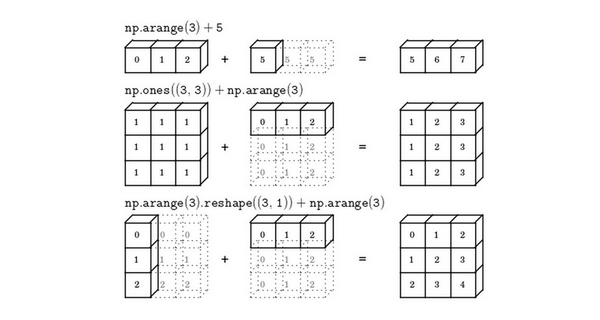星期四, 二月 13日 2020, 5:22 下午

2.1k 字     8 分钟

# 向量与矩阵

import numpy as np #导入numpy库
a=np.random.randn(5)
print(a)
print(a.shape)
print(a.T)
print(np.dot(a,a.T))


[ 1.38747875  1.05981906 -0.41137174  0.85581765  0.25313936]
(5,)
[ 1.38747875  1.05981906 -0.41137174  0.85581765  0.25313936]
4.014043815271083


import numpy as np #导入numpy库
a=np.random.randn(5,1)
print(a)
print(a.shape)
print(a.T)
print(np.dot(a,a.T))


 [ 0.14745407]
[ 0.04916029]
[-0.78656516]
[ 1.37759773]]
(5, 1)
[[-0.28745951  0.14745407  0.04916029 -0.78656516  1.37759773]]
[[ 0.08263297 -0.04238707 -0.01413159  0.22610564 -0.39600357]
[-0.04238707  0.0217427   0.00724889 -0.11598223  0.20313239]
[-0.01413159  0.00724889  0.00241673 -0.03866777  0.06772311]
[ 0.22610564 -0.11598223 -0.03866777  0.61868476 -1.08357039]
[-0.39600357  0.20313239  0.06772311 -1.08357039  1.89777551]]


from numpy import *

# 数组
v1 = array([1, 0, 1])
v11 = array([1, 1, 1])

# 2*3矩阵
v2 = array([[1, 1, 2], [1, 1, 0]])

# 线性代数矩阵乘法，行乘列，再相加
print("一维向量dot： ", dot(v11, v1))
print("一维dot二维： ", dot(v2, v1))


一维向量dot：  2



A = np.array([1,2,3])
result = A + 100
print(result)


[101 102 103]


A = np.array([[1,2,3,5],[1,2,3,5],[1,2,3,5]])
cal = np.array([100,1,1,1])
result = A/cal
print(result)


[[0.01 2.   3.   5.  ]
[0.01 2.   3.   5.  ]
[0.01 2.   3.   5.  ]]


A = np.array([[1,2,3,5],[1,2,3,5],[1,2,3,5]])
cal = np.array([100,1,1,1])
result = A/cal.reshape(1,4)
print(result)


A/cal.reshape(1,4)指令则调用了numpy中的广播机制。这里使用 $3 \times 4$的矩阵$A$除以 $1 \times 4$的矩阵$cal$。技术上来讲，其实并不需要再将矩阵$cal$ reshape(重塑)成 $1 \times 4$，因为矩阵$cal$本身已经是 $1 \times 4$了。但是当我们写代码时不确定矩阵维度的时候，通常会对矩阵进行重塑来确保得到我们想要的列向量或行向量。重塑操作reshape是一个常量时间的操作，时间复杂度是$O(1)$，它的调用代价极低。"觉得赞的话，微信扫一扫赞赏一下吧"

Plugins